48-hour FLASH SALE: Get 30% Off All Certificates and Diplomas! Hurry, sale ends on Thursday, 12th December 2019.### Ce cours a été révisé!

Pour une expérience d'apprentissage plus agréable, nous vous recommandons d'étudier la version mobile relogée de ce cours.

Emmenez-moi au cours révisé.

- or -

Continue studying this course

# Amortissement

• Notes d'étude
• Révisions du sujet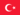BabaJide Martins F. 0 0 Decrease in value due to wear and tear, decay, decline in price. Accumulated depreciation must be deducted and posted to balance sheet.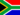Morne V. 0 0 'Accumulated depreciation' account, which is posted to the Balance sheet as a deduction from the relevant non-current asset.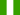Sunday O. 0 0 Depreciation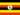Odongo M. 0 0 Accounting -> Depreciation Depreciation Depreciation is defined as the allocation of the cost of a non-current asset over the economic life of that asset. It is charged at the end of each accounting period. At the end of each accounting period depreciation accumulates and it is the 'Accumulated depreciation' account, which is posted to the Balance sheet as a deduction from the relevant non-current asset. When preparing to tackle the question, the following points must be ascertained: the cost of the asset the life of the asset the nature of use, revenue pattern the residual value of the assetSaul K. 0 0 can buildings depprecite?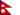Manish K. 0 0 chapter is clearly understood. Depreciation is deducted from non-current assets to find out actual value of assets right now. Different methods are used to calculate depreciation.Penelope M. 0 0 I see the value of the car which is at \$13500, as soon as you drive the vehicle off the lot the value is gone down.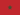Adil N. 0 0 What does depreciation mean?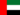Gloria N. 0 0 Depreciation is defined as the allocation of the cost of a non-current asset over the economic life of that asset.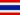Epie E. 0 0 Assets are depriciated based on theirs cost,function(nature of its use) to farch revenue.And we deduct accumulated depreciation from non current assets to get the true value of the asset in question.
• Text Version